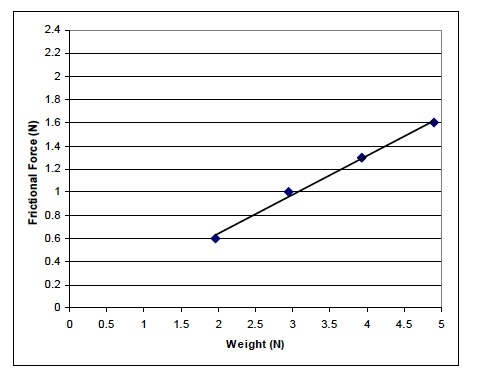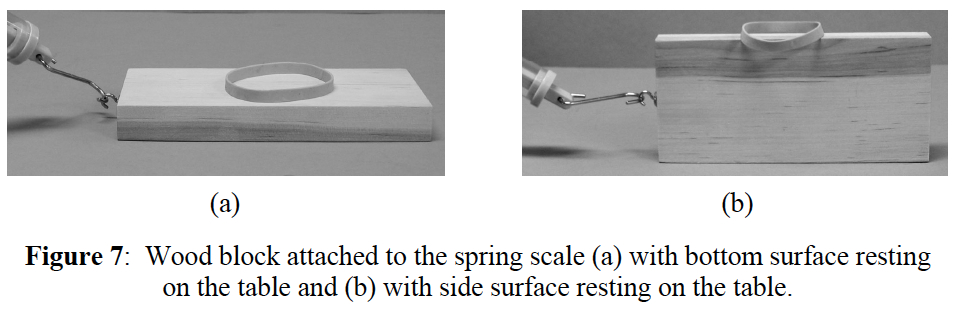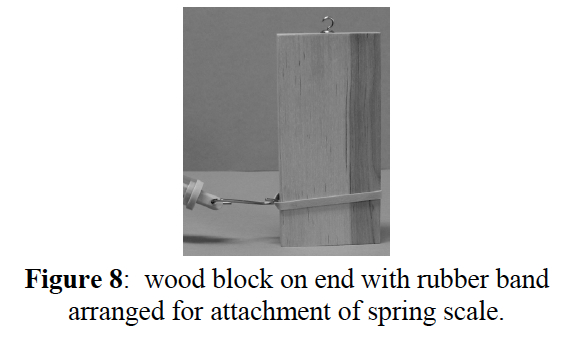# Lab### MINDSET

This Investigation is designed to:

demonstrate to you that the frictional force between an object and a surface depends on the weight of the object,

demonstrate to you that the frictional force between an object and a surface is independent of the surface area of the object

use graphs to illustrate the relationships between frictional force and weight and frictional force and surface area.

### BE PREPARED

Student Preparation for the Investigation includes having students gather the following materials. This preparation takes place on lab day after student lab groups have settled at their assigned lab tables.

Note: The materials are listed in students’ SDRs. They are also listed below for your reference.

(1) wood box with hook

(1) woodblock with hook

(3) 100 g weights

(1) 5 N spring scale

(1) triple beam balance

(1) metric ruler

(1) calculator

(1) roll of masking tape (optional)

(1) rubber band

(1) meter stick (optional)

Direct one student from each lab group to collect the materials listed in their SDRs.

### INVESTIGATE

If you were unable to leave your pieces of tape on the table, set up your lab bench as in Investigations One and Two, using four pieces of tape to create a starting line and a finish line. Remember, the lines should be 50 cm apart.

The first variable you will investigate is weight. Predict whether or not the weight of the wood box will affect the frictional force between it and the table. Record your predictions in your Investigation Three Data Record.

Before beginning the experiment, identify the independent and dependent variables in this experiment.

Reflect on the PreLab video as you move through the procedural steps.

Explain to students that during the Experiment, every procedural step is important. If one step is skipped, data can become invalid. To help you keep on track, read each step thoroughly, complete the step, then check it off (Read it – Do it – Check it).

Complete all of the procedural steps in your SDR.

Note: The procedural steps are listed below for your reference.

Trial 1

Determine the weight of each load listed in Table A.

To find the weight, you must first find the mass of each object.

The mass of each 100 g weight is 100 g.

Measure the mass of the woodblock using the triple beam balance.

Record: Write the mass of each load in Table A.

Calculate: Determine the mass of each load.

Convert mass in grams to kilograms.

This is done by dividing grams by 1000.

grams ÷ 1000 = kilograms

200.7 g (woodblock) ÷ 1000 = 0.2007 kg

Record: Write the mass in kilograms in Table A.

Calculate: Determine the weight of each load.

Use the following formula:

Weight (N) = mass (kg) × acceleration due to gravity (m/s2)

Weight = 0.2007 kg × 9.8 m/s2

Weight = 1.97 N

Record: Write your answers in Table A.

Begin the trials.

Pull each load from 20 cm behind the starting line to 20 cm past the finish line.

Read the spring scale as the load is pulled.

Remember to keep a constant velocity between the two lines.

Record: Use the spring scale to determine the amount of frictional force between the load and the table. Record the value in Table A.

Note: Although the velocity at which you pull the load is not important in this experiment, you must pull the load at a constant velocity.

Note: As one student is pulling the load, another student should read the spring scale.

Briefly review the results from Table A as a class.

Did the amount of frictional force between the table and each load differ as the weight of the load changed?

Graph: Use a grid similar to the one below to plot the data.

Determine which variable is the independent variable and which is the dependent variable.

Draw a best fit line. If you need help, refer to the procedure, Drawing a Best Fit LineBriefly review the graph as a class.

Describe the relationship between weight and frictional force based on your graph.

Trial 2

The second variable you will investigate is surface area.

Predict whether or not the surface area of the woodblock will affect the frictional force between it and the table.

Record your predictions in Trial 2 of your Investigation Three Data Record.

You will pull the block across the table in three ways.

You will pull it so that the larger bottom surface rests on the table (Figure 7a).

You will pull it so that the smaller side surface rests on the table (Figure 7b).

You will pull it so that the end surface rests on the table (Figure 8).

Note: A rubber band will be needed to hook the spring scale when pulling the woodblock on its end surface. Therefore, the rubber band must be added when the woodblock is pulled on its bottom and side surfaces, as shown in Figures a and b. The rubber band must be added to each load so that the mass of the load is equalized.Calculate: Determine the surface area (cm2) of each of the three surfaces.

Use the following formula: Surface area (cm2) = length (cm) × width (cm)

Record: Write your answer in Table B.

Begin the trials.

1. Pull each load from 20 cm behind the starting line to 20 cm past the finish line.
2. Read the spring scale as the load is pulled.
3. Remember to keep a constant velocity between the two lines.
4. Record: Obtain the data for surface area from Table C and write it in the second column of Table D.
5. Record: Use the spring scale to determine the amount of frictional force between the load and the table. Record the value in Table C.Briefly review the results as a class.

• Did the amount of frictional force between the table and the block differed as the surface area in contact with the table changed?

### CLEAN UP

Be sure to clean up your lab bench after finishing the experiment.